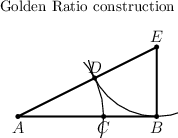Golden ratio

golden section, sectio aurea, sectio divina, divina proportio, golden mean, harmonic division, division in extreme and mean ratio

The division of a line segment $a$ into two parts the greater of which, $x$, is the mean proportional between the whole segment $a$ and the smaller part $a-x$, i.e. $\label{ratio} a : x = x : (a-x).$

To find $x$, one has to solve a quadratic equation, $x^2+ax-a^2 = 0,$ the positive solution of which is

$x = \frac{-1+\sqrt{5}}{2} a \approx 0.62 a.$

Condition \eqref{ratio} may also be written as

$\frac{x}{a}\left(1+\frac{x}{a}\right) = 1 \quad \text{or} \quad x = \frac{a}{1+\frac{x}{a}}$

or $x = a \frac{ 1}{ 1+ \frac{1}{1 + \frac{1}{1 + \frac{1}{1 + \ldots}}} },$

i.e. representing $x$ as a continued fraction, the convergents of which are

$\frac{1}{1}, \frac{1}{2}, \frac{2}{3}, \frac{3}{5}, \frac{5}{8}, \frac{8}{13}, \frac{13}{21}, \text{etc.},$

where $1,1,2,3,5,8,13,21,\ldots,$ are the Fibonacci numbers.The golden ratio of a segment $AB$ may be found by a geometric construction (see Fig.). Draw the perpendicular to $AB$ at $B$; on the perpendicular, mark off the segment $BE=AB/2$; then join $A$ and $E$, mark off $ED=EB$ and finally $AC=AD$. Then

$AB:AC=AC:CB .$

The golden ratio was already known in Antiquity. Its first appearance in the extant classical literature is in Euclid's Elements, Book II, 11 (3th century B.C.).

Principles based on the golden ratio or similar proportional ratios provided the basis for the compositional design of many works of art (mainly architectural works of Antiquity and the Renaissance).